# Mensuration solutions class 10 Maths SSC Exercise 10.1,10.2,10.3 and 10.4

SSC maths Mensurations solutions for class 10 exercise 10.1, 10.2, 10.3 and 10.4 are given.

Study the text book lesson Mensuration very well.

Observe the example problems and solutions given in the textbook.

The given solutions are very easy to understand.

Observe the solutions given below and try them in your own method.

You can see the text book solutions for

2. Sets

10. Mensuration

11. Trigonometry

13. Probability

14. Statistics

# SSC maths class 10 Mensuration solutions

Chapter 10

Mensuration

Exercise 10.1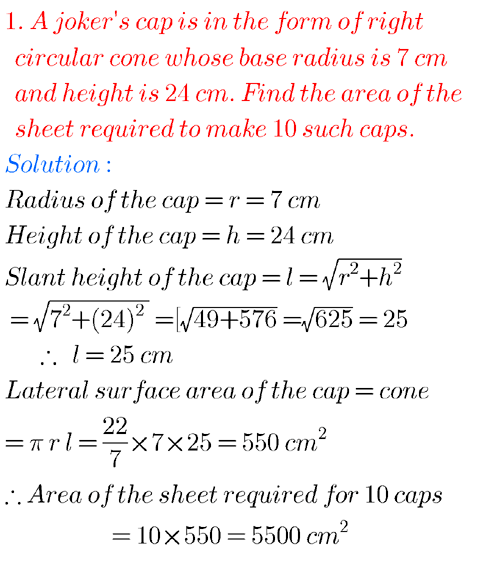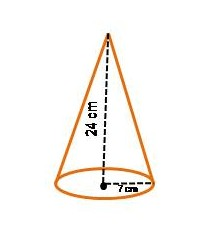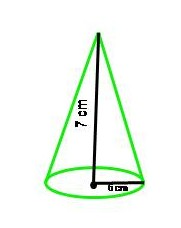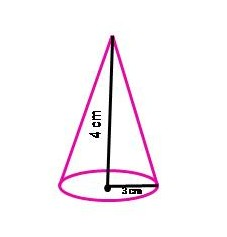## Tenth maths guide for maths mensuration ssc

Exercise 10.2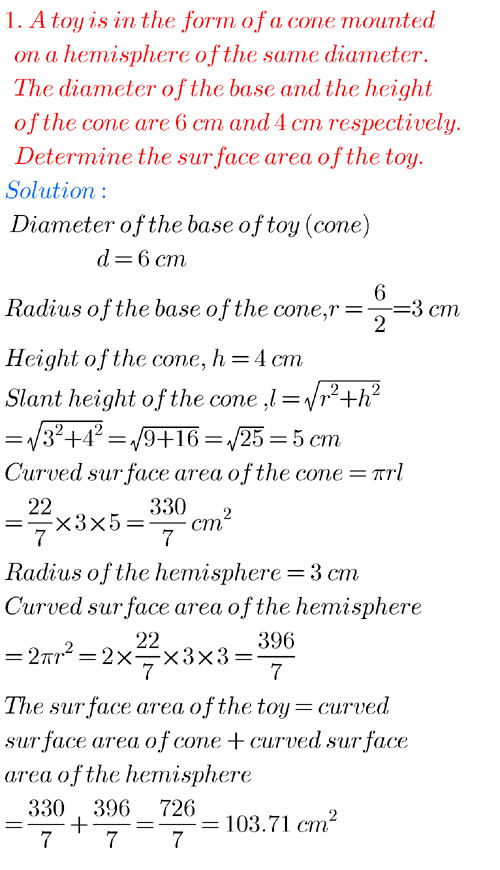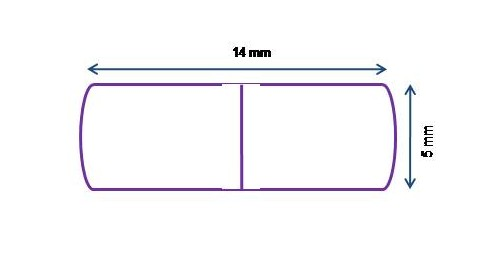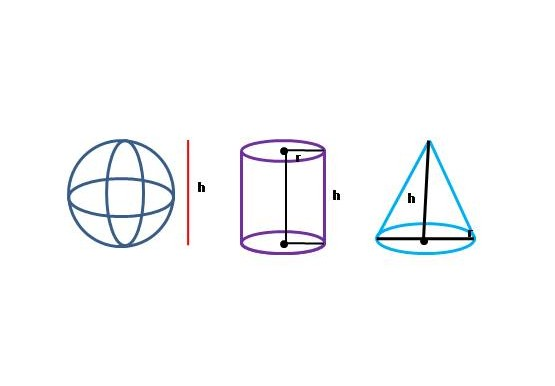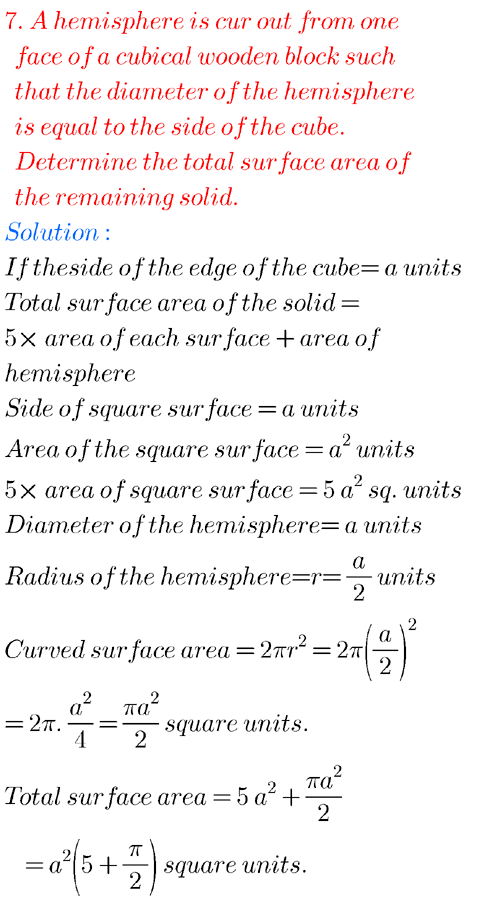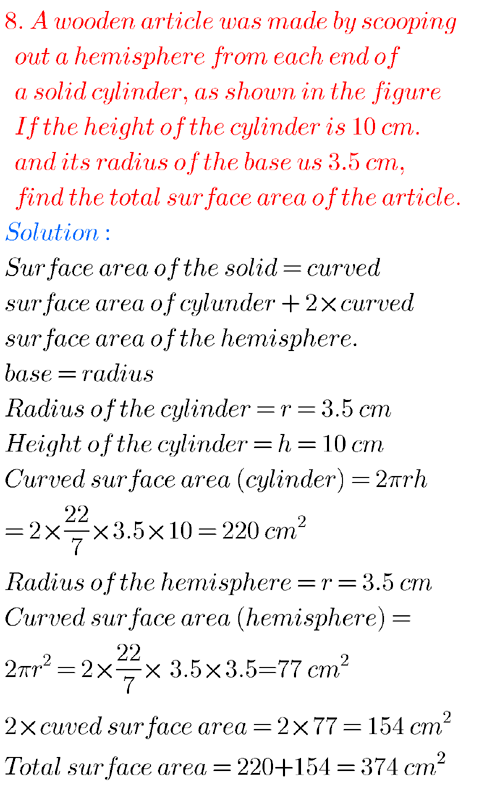## Class X maths solutions for mensuration

Exercise 10.3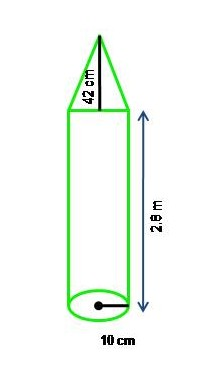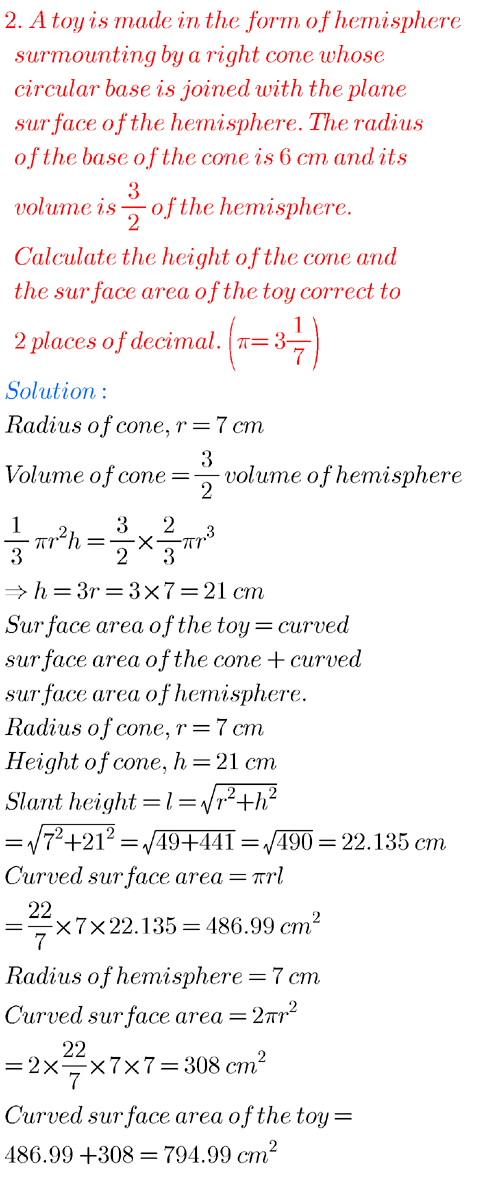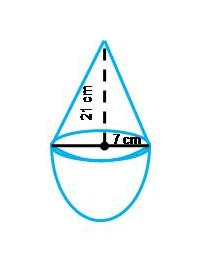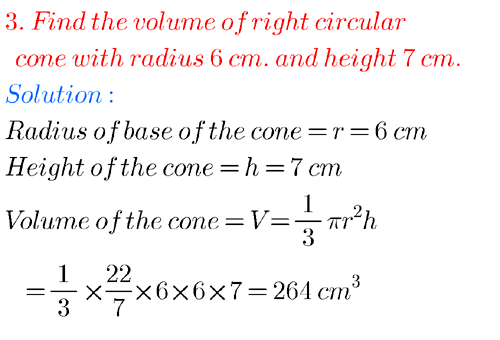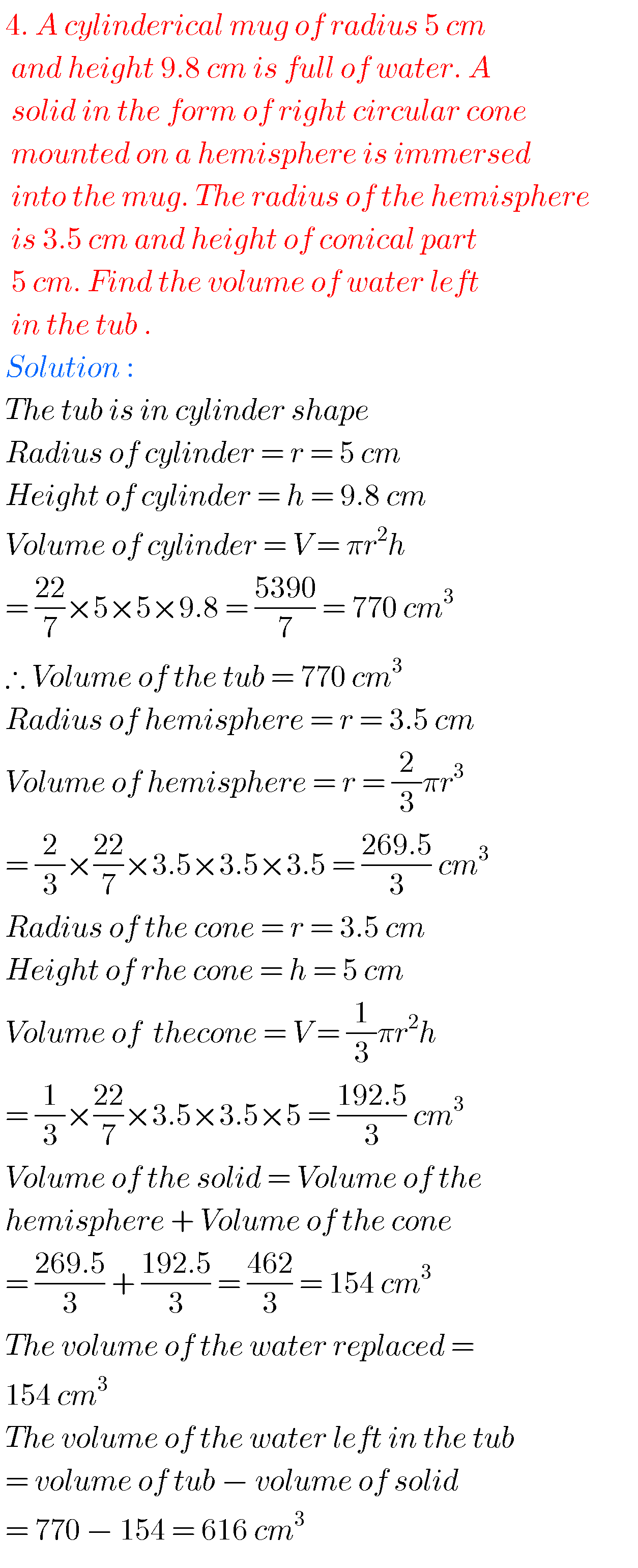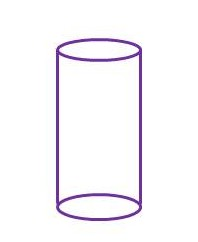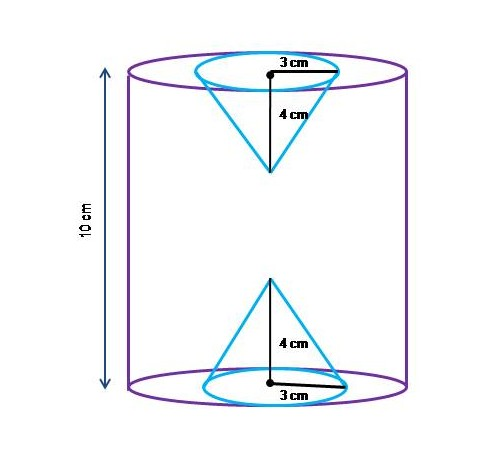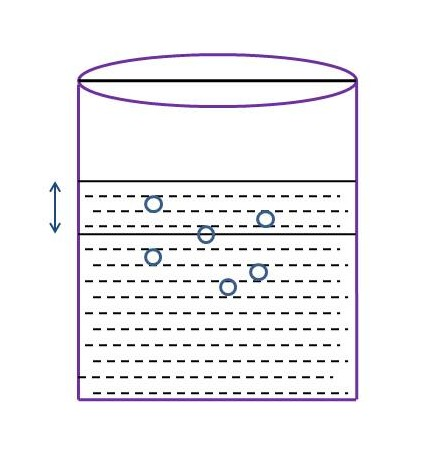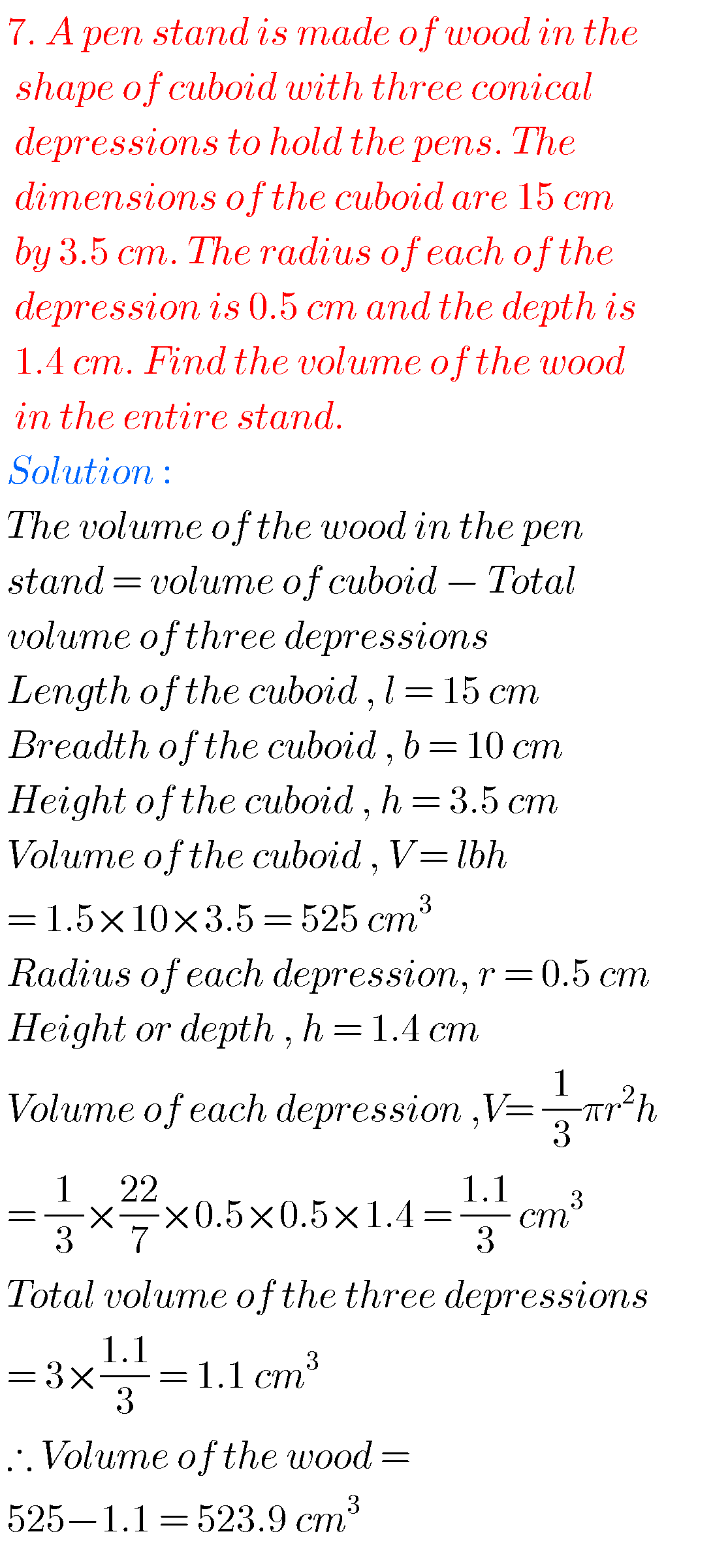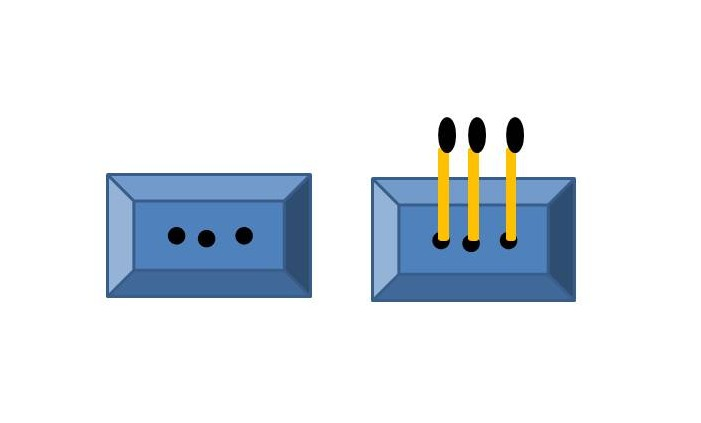### 10th maths mensuration solutions

Exercise 10.4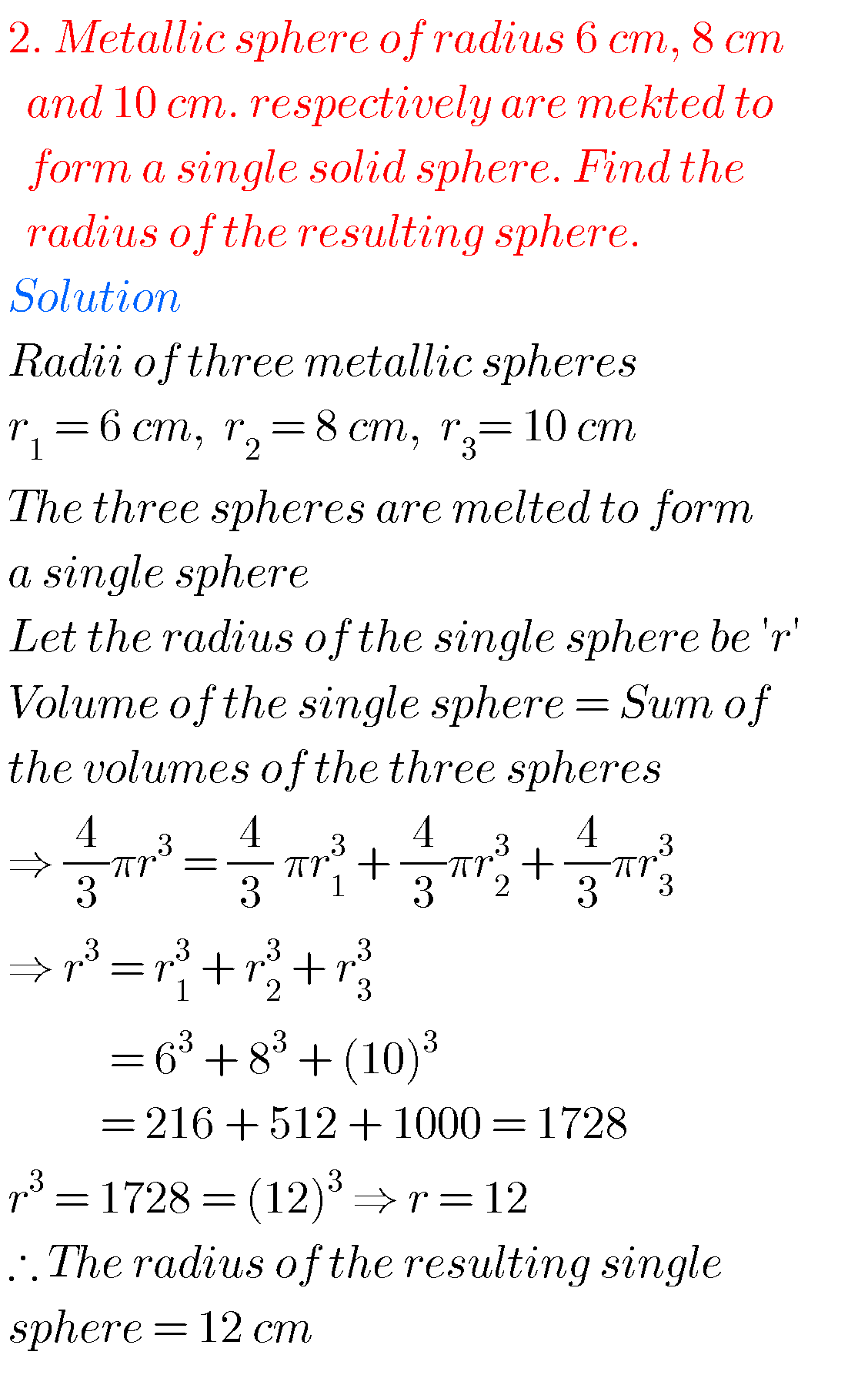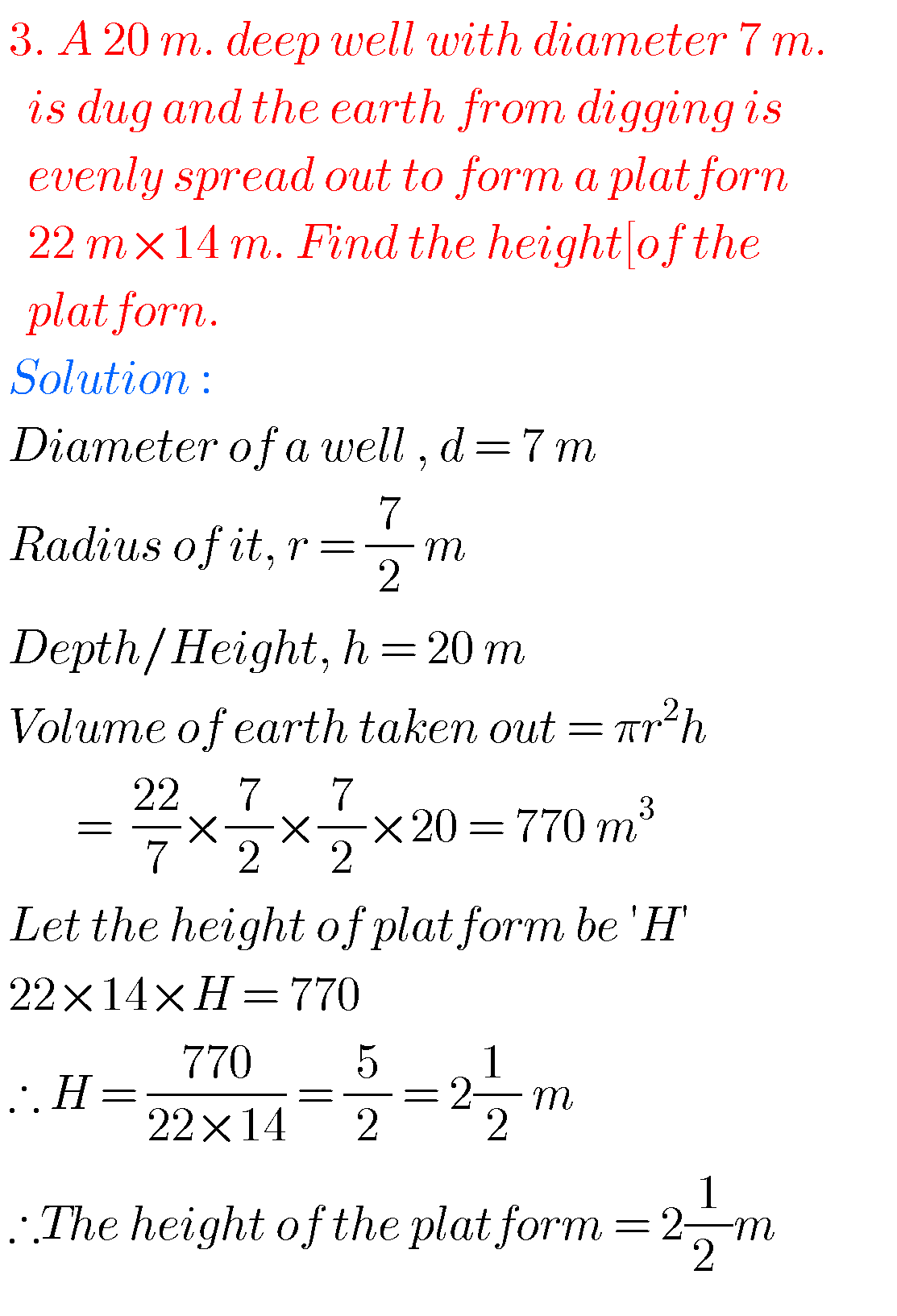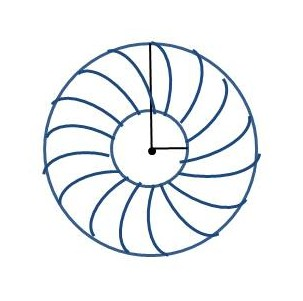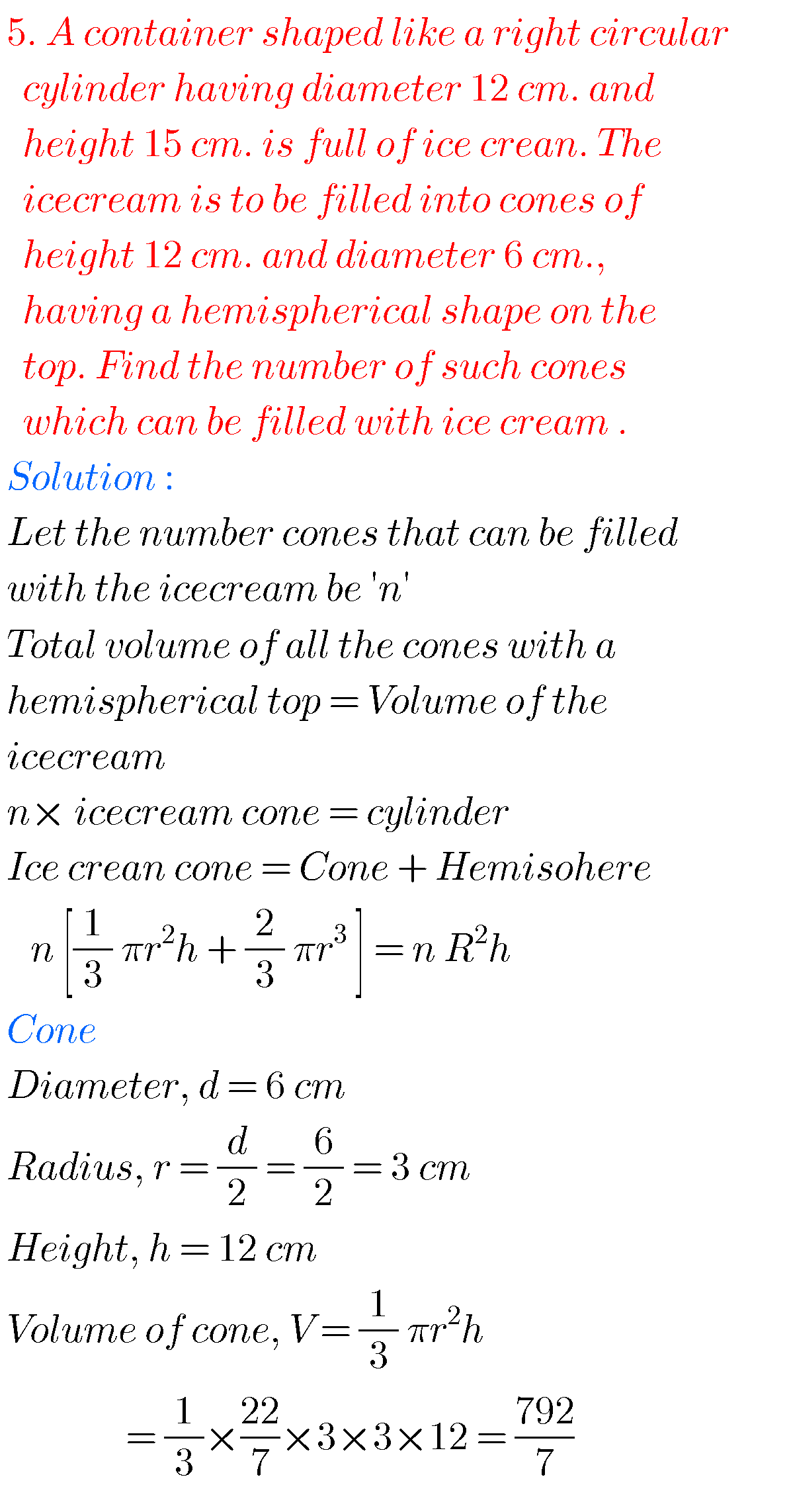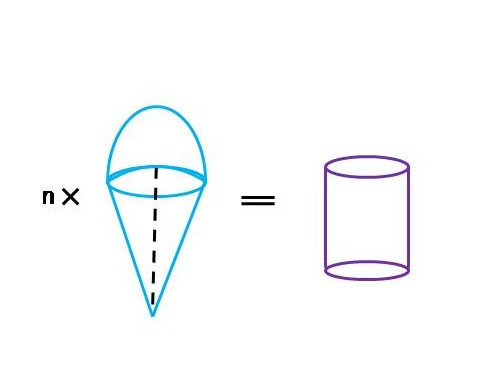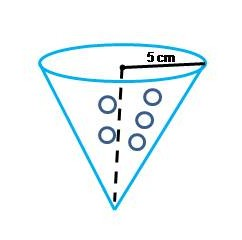Optional Exercise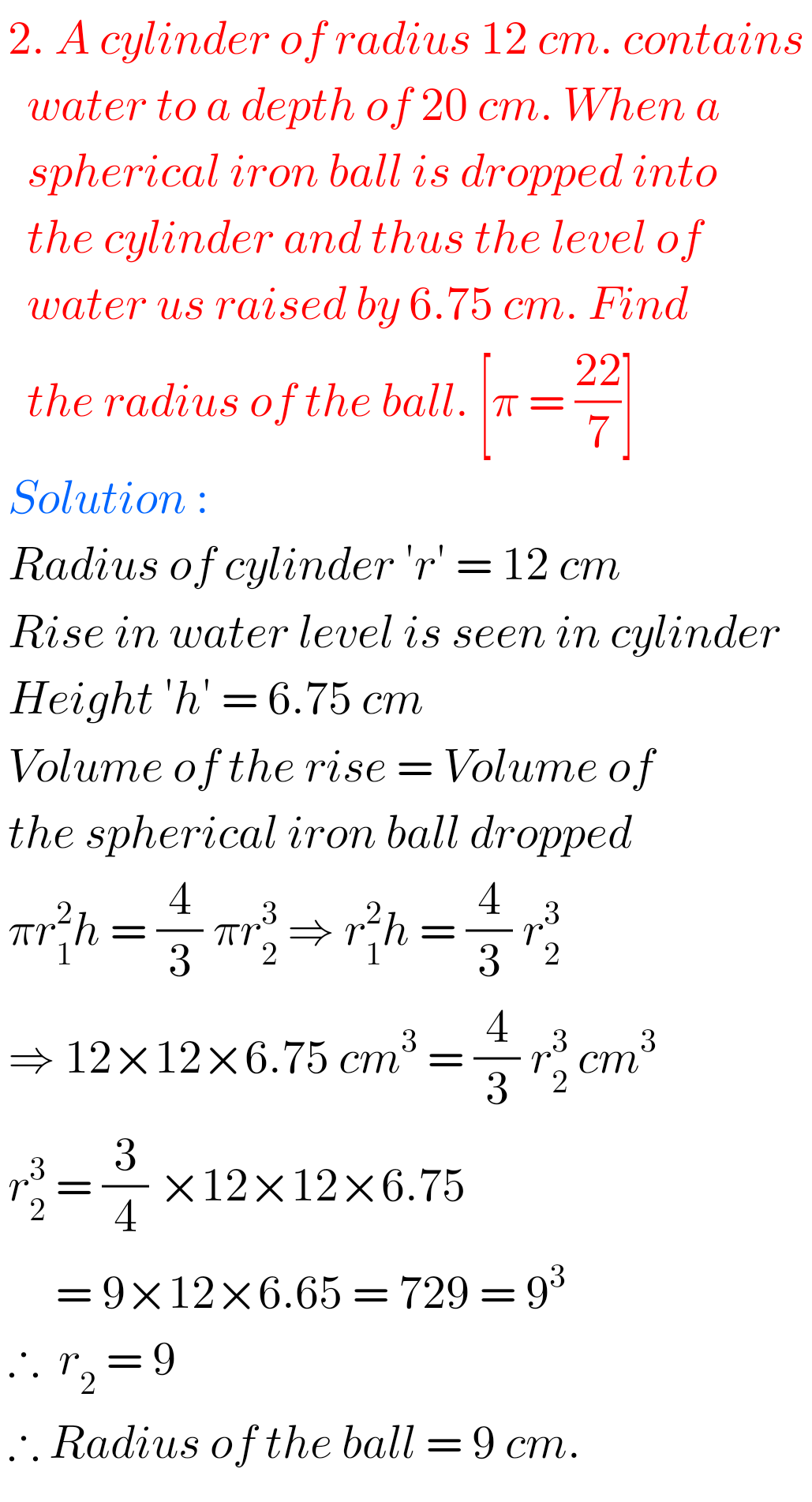3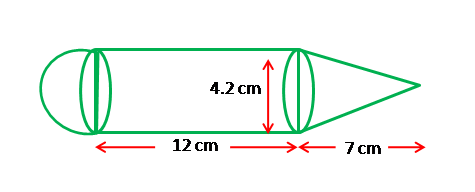Note :

Observe the solutions and try them in your own methods.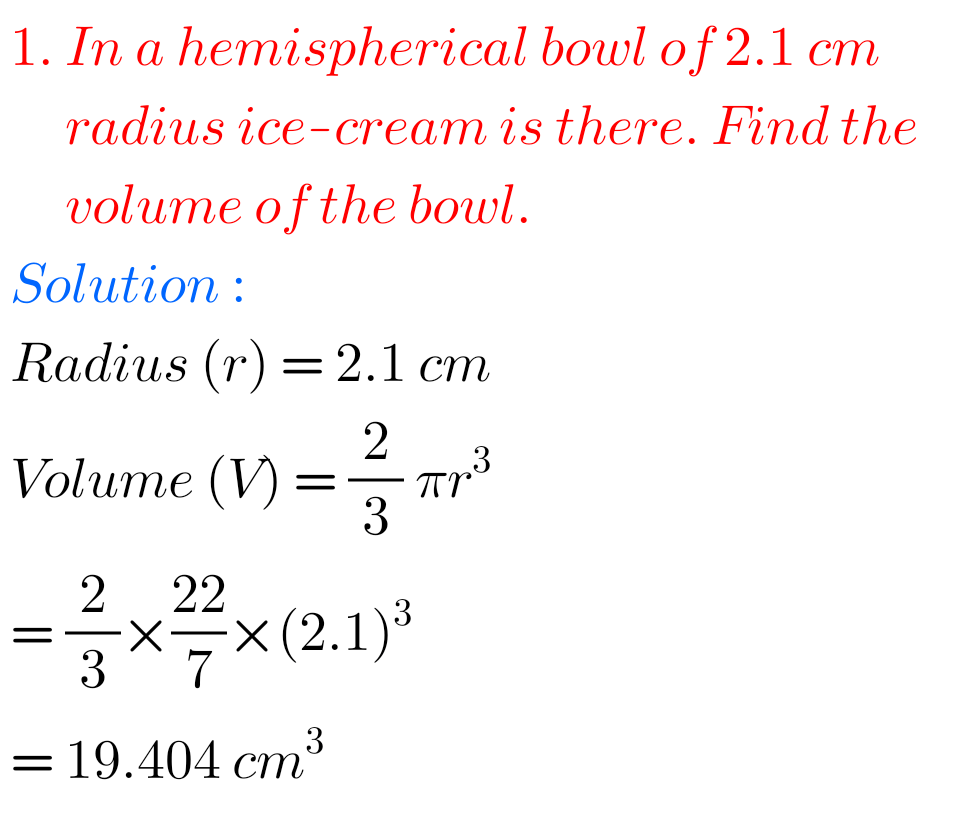Inter maths solutions for 1a for exam purpose

Inter maths 1b solutions for exam purpose

Ncert maths class 7 fractions and decimals

Ncert maths class 6 playing with numbers

Ncert solutions for maths class 7 rational numbers

Ncert solutions for maths class 6 knowing our numbers

Ncert solutions for maths class 6 integers

Ncert solutions for maths class 7 integers

### 6 thoughts on “Mensuration solutions class 10 Maths SSC”

1.It was very useful for me .Thank you very much for everyone who has worked for it .

2.Thank u very much for ur helping

3.Karthik chowdary

Thank you all for this who worked on this😊❤️❤️❤️ it’s very helpful to me ☺️

4.Because of mathsglow.com I completed my notes.
I am very thankful to mathsglow.
Every student needs It.

5.Thank u very much for your helping.
This is very useful to student .
Every student needs it.

6.Thank you all for this who worked on this😊❤️❤️❤️ it’s very helpful to me and all 😉